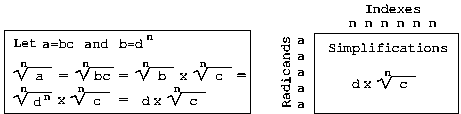### Chart of Interesting Radicands (<300) for the Nth Root (Indexes 2-10) Simplification of Whole NumbersExplanation:

Typical nth root problems say things like: simply the square root of 162.
Solving might look like:
square root of 162 = square root of 2*81
square root of 2*81= square root of 2*9*9
square root of 2*9*9 = square root of 2*3*3*3*3
square root of 2*3*3*3*3 = square root of 2*(3*3)*(3*3)
square root of 2*(3*3)*(3*3) = 3 times the square root of 2*(3*3)
3 times the square root of 2*(3*3) = 3*3 times the square root of 2
3*3 times the square root of 2 = 9 times the square root of 2

This is a chart so you can "look up" the answers instead of calculate them (and also just for random nth root comparisons).

So for the above problem, you scroll down to 162 (the radicand is the part under the square root symbol). You look in the 2's column (the index is the nth root so 2 is a square root, 3 is a cube root, 4 is a 4th root, and so on). And there's the answer 9*sqrt(2).

If the number you are trying to simplify is greater than 1 and is less than 300 and has an index less than 10 AND it is not in this chart, it doesn't simplify any more (2, 3, 7, 10, and so on). Only whole number answers are provided for radicands greater than 300.

 Index 2 3 4 5 6 7 8 9 10 Radicand 4 2 8 2*sqrt(2) 2 9 3 12 2*sqrt(3) 16 4 2*cbrt(2) 2 18 3*sqrt(2) Index 2 3 4 5 6 7 8 9 10 20 2*sqrt(5) 24 2*sqrt(6) 2*cbrt(3) 25 5 27 3*sqrt(3) 3 28 2*sqrt(7) 32 4*sqrt(2) 2*cbrt(4) 2*4thrt(2) 2 36 6 Index 2 3 4 5 6 7 8 9 10 40 2*sqrt(10) 2*cbrt(5) 44 2*sqrt(11) 45 3*sqrt(5) 48 4*sqrt(3) 2*cbrt(6) 2*4thrt(3) 49 7 50 5*sqrt(2) 52 2*sqrt(13) 54 3*sqrt(6) 3*cbrt(2) 56 2*sqrt(14) 2*cbrt(7) Index 2 3 4 5 6 7 8 9 10 60 2*sqrt(15) 63 3*sqrt(7) 64 8 4 2*4thrt(4) 2*5thrt(2) 2 68 2*sqrt(17) 72 6*sqrt(2) 2*cbrt(9) 75 5*sqrt(3) 76 2*sqrt(19) Index 2 3 4 5 6 7 8 9 10 80 4*sqrt(5) 2*cbrt(10) 2*4thrt(5) 81 9 3*cbrt(3) 3 84 2*sqrt(21) 88 2*sqrt(22) 2*cbrt(11) 90 3*sqrt(10) 92 2*sqrt(23) 96 4*sqrt(6) 2*cbrt(12) 2*4thrt(6) 2*5thrt(3) 98 7*sqrt(2) 99 3*sqrt(11) Index 2 3 4 5 6 7 8 9 10 100 10 104 2*sqrt(26) 2*cbrt(13) 108 6*sqrt(3) 3*cbrt(4) 112 4*sqrt(7) 2*cbrt(14) 2*4thrt(7) 116 2*sqrt(29) 117 3*sqrt(13) Index 2 3 4 5 6 7 8 9 10 120 2*sqrt(30) 2*cbrt(15) 121 11 124 2*sqrt(31) 125 5*sqrt(5) 5 126 3*sqrt(14) 128 8*sqrt(2) 4*cbrt(2) 2*4thrt(8) 2*5thrt(4) 2*6thrt(2) 2 132 2*sqrt(33) 135 3*sqrt(15) 3*cbrt(5) 136 2*sqrt(34) 2*cbrt(17) Index 2 3 4 5 6 7 8 9 10 140 2*sqrt(35) 144 12 2*cbrt(18) 2*4thrt(9) 147 7*sqrt(3) 148 2*sqrt(37) 150 5*sqrt(6) 152 2*sqrt(38) 2*cbrt(19) 153 3*sqrt(17) 156 2*sqrt(39) Index 2 3 4 5 6 7 8 9 10 160 4*sqrt(10) 2*cbrt(20) 2*4thrt(10) 2*5thrt(5) 162 9*sqrt(2) 3*cbrt(6) 3*4thrt(2) 164 2*sqrt(41) 168 2*sqrt(42) 2*cbrt(21) 169 13 171 3*sqrt(19) 172 2*sqrt(43) 175 5*sqrt(7) 176 4*sqrt(11) 2*cbrt(22) 2*4thrt(11) Index 2 3 4 5 6 7 8 9 10 180 6*sqrt(5) 184 2*sqrt(46) 2*cbrt(23) 188 2*sqrt(47) 189 3*sqrt(21) 3*cbrt(7) 192 8*sqrt(3) 4*cbrt(3) 2*4thrt(12) 2*5thrt(6) 2*6thrt(3) 196 14 198 3*sqrt(22) Index 2 3 4 5 6 7 8 9 10 200 10*sqrt(2) 2*cbrt(25) 204 2*sqrt(51) 207 3*sqrt(23) 208 4*sqrt(13) 2*cbrt(26) 2*4thrt(13) 212 2*sqrt(53) 216 6*sqrt(6) 6 Index 2 3 4 5 6 7 8 9 10 220 2*sqrt(55) 224 4*sqrt(14) 2*cbrt(28) 2*4thrt(14) 2*5thrt(7) 225 15 228 2*sqrt(57) 232 2*sqrt(58) 2*cbrt(29) 234 3*sqrt(26) 236 2*sqrt(59) Index 2 3 4 5 6 7 8 9 10 240 4*sqrt(15) 2*cbrt(30) 2*4thrt(15) 242 11*sqrt(2) 243 9*sqrt(3) 3*cbrt(9) 3*4thrt(3) 3 244 2*sqrt(61) 245 7*sqrt(5) 248 2*sqrt(62) 2*cbrt(31) 250 5*sqrt(10) 5*cbrt(2) 252 6*sqrt(7) 256 16 4*cbrt(4) 4 2*5thrt(8) 2*6thrt(4) 2*7thrt(2) 2 Index 2 3 4 5 6 7 8 9 10 260 2*sqrt(65) 261 3*sqrt(29) 264 2*sqrt(66) 2*cbrt(33) 268 2*sqrt(67) 270 3*sqrt(30) 3*cbrt(10) 272 4*sqrt(17) 2*cbrt(34) 2*4thrt(17) 275 5*sqrt(11) 276 2*sqrt(69) 279 3*sqrt(31) Index 2 3 4 5 6 7 8 9 10 280 2*sqrt(70) 2*cbrt(35) 284 2*sqrt(71) 288 12*sqrt(2) 2*cbrt(36) 2*4thrt(18) 2*5thrt(9) 289 17 292 2*sqrt(73) 294 7*sqrt(6) 296 2*sqrt(74) 2*cbrt(37) 297 3*sqrt(33) 3*cbrt(11) 300 10*sqrt(3) Index 2 3 4 5 6 7 8 9 10 343 7 512 8 2 625 25 5 729 9 3 1000 10 1024 4 2 Index 2 3 4 5 6 7 8 9 10

Note that you can also "reverse": See the 3 halfway between the two "index" 6's above? That's 3 raised to the 6th power equals the leftmost column, 729, as well as 93. So 36 = 93 = 729.

Using the above chart, which numbers below 100 are you most likely to see as radicands in simplification problems? What "rules" for exponents (the indexes) do you see?

### Another way to look up some common radicands for square and cube roots.

These two grids work just like the original multiplication grids (side * top = center). The sorted numbers on right are the radicands that appear in the grid. This isn't like looking for numbers on a bingo card. If you don't see your number to the right, it's not in the grid and maybe you should look in the above chart instead.Did you notice that the radicand of the top label row multiplied by the radicand in the 1's column is the radicand where two meet up (horizontally and vertically)? Using that, what belongs in the space for 5 times the cubed root of 3?

Do you see errors on this page? If so, let me know!